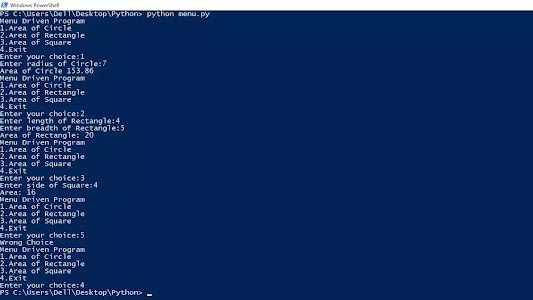# Menu Driven Program in Python using while loop

Menu-driven program in Python: A program that obtains choice from a user by displaying the menu.

## Menu Driven Program in Python using while loop

In this post, we are going to make a menu-driven program in python using while loop and simple if-else statements for the following operations:
1. Area of Circle
2. Area of Rectangle
3. Area of square
4. Exit

## Menu-driven program in Python class 11

```while True:
print("1.Area of Circle")
print("2.Area of Rectangle")
print("3.Area of Square")
print("4.Exit")
if choice==1:

elif choice==2:
length=int(input("Enter length of Rectangle:"))

elif choice==3:
side=int(input("Enter side of Square:"))
print("Area:",side*side)
elif choice==4:
break
else:
print("Wrong Choice")```

Quick algorithm for Python Menu-driven Program:
• Print the menu on the screen.
• Input the choice from the user.
• According to his/her choice, display the area using the if-else statement.
For ex:
if choice==1:Related posts:

## Menu Driven Program in Python using functions

In this program, we are going to create a menu-driven program in python for class 12 in which we will calculate the area of different shapes like circle, square, and rectangle using functions.
```def circle(radius):

def square(side):
print("Area:",side*side)

while True:
print("1.Area of Circle")
print("2.Area of Rectangle")
print("3.Area of Square")
print("4.Exit")

if choice==1:

elif choice==2:
length=int(input("Enter length of Rectangle:"))

elif choice==3:
side=int(input("Enter side of Square:"))
square(side)

elif choice==4:
break
else:
print("Wrong Choice")```

This Menu-driven program in python using functions is very important for class 12th students. One question of this program can come in CBSE board, so prepare well for it. If, you have any doubt let me know in the comment section.

We can also call this program python menu loop because we will display the menu in a loop until the user wants to exit.
Related post:• Hackerrank 5* • Full Stack Developer • Masters in SEO

### Related Posts

1.how can we make the loop run again to accept the input if input is "Wrong Choice"
while True:
dine=eval(input("Please type 1 for Drive-Thru ; 2 for Dine-in ; 3 for Carry-out/Delivery"))

if dine==1:
dine_type="Drive-Thru"

elif dine==2:
dine_type="Dine-in"

elif dine==3:
dine_type="Carry-out/Delivery"
else:
print("Wrong Choice")

2.is def compulsary for structure progamming?

1.No, it's not compulsory to use functions for structure programming.

We break complex programs into small tasks using functions.

To organize our programs in python we use functions.